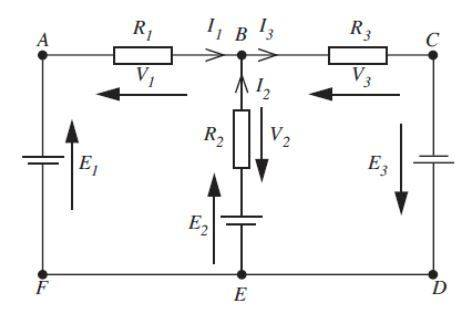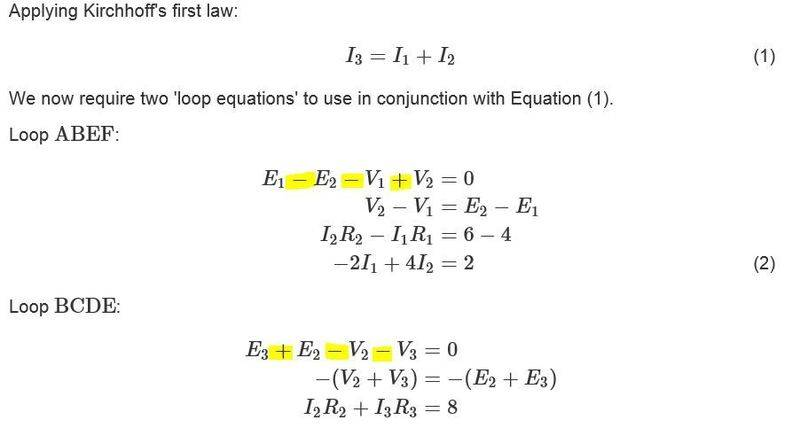# Can anyone explain the order of these signs please?## Homework Statement

Create two loop equations.

I3=I1+I2

## The Attempt at a Solution

The solution is given but I do not understand why the signs are ordered as they are.

I understand that in KCL the sum of the currents flowing towards the junction are equal to the sum of the currents moving away.

I also understand that in KVL the sum of the voltage drops is equal to zero.

The example combines these two methods and then goes on to solve by simultaneous equations which I can follow ok.

My problem is with the initial order of the signs (highlighted in yellow). Can anyone explain these please?
My text books and examples online seem to approach it slightly differently and I just can't figure it out.
I'm going round and round with it, getting nowhere.
I've just added the images to the post as I see they are difficult to deal with the other way.

Last edited:
•the student

•